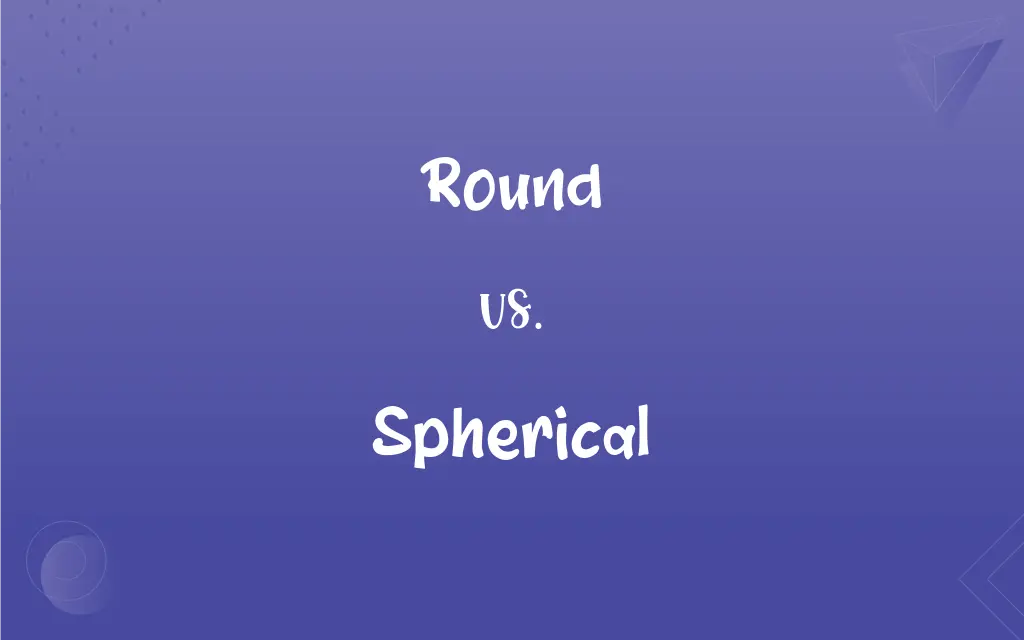# Round vs. Spherical: What's the Difference?Edited by Huma Saeed || By Sumera Saeed || Updated on November 6, 2023
Round describes a shape that is circular in two dimensions, while spherical refers to a three-dimensional shape where all points are equidistant from the center.## Key Differences

Round is a term that can describe objects with a circular shape or form, typically in a two-dimensional context, like a round plate. The word can also refer to shapes that are somewhat circular or curved without being perfectly circular. Spherical, however, specifically describes a three-dimensional form that is perfectly round in all directions, like a globe, with every surface point equidistant from the center.
The term round can apply to shapes and objects in everyday contexts, such as a round table or a round coin, which are circular when viewed from above but flat or not fully three-dimensional. Spherical is more precise and scientific, used to describe objects like planets or balls where symmetry is observed from the center to any point on its surface, not just in a single plane.
Round does not necessarily mean perfectly circular; it can be used to describe things that approximate a circle. For instance, a round face may not be a perfect circle, but it gives the general impression of roundness. Spherical, on the other hand, implies a stricter geometry, where all cross-sections through the center would yield perfect circles, such as in the case of a basketball.
In everyday language, round is a more common and less technical term, which can also be used figuratively to suggest completeness or entirety, as in "round-the-clock" service. Spherical is less likely to be used in a non-literal sense and conveys no meaning beyond its geometric implications, stressing the three-dimensional aspect of roundness in a literal sense.
When considering objects in the physical world, round objects can include flat circles, cylinders, or even ovals, reflecting a broader range of shapes. In contrast, spherical objects have a strict definition in geometry, maintaining the same distance from the center to the surface throughout, resulting in a perfect ball shape.

## Comparison Chart

### Dimensionality

Two-dimensional or approximating a circle in 3D.
Three-dimensional and perfectly round.

### Geometric Precision

Can be less precise, often circular but not always.
Always geometrically precise and equidistant.

### Common Usage

Used for everyday objects, can be figurative.
Used for geometrically perfect objects, rarely figurative.

### Implication of Completeness

Can imply wholeness or completeness.
Does not imply completeness, only shape.

### Example Objects

Plates, coins, full moons.
Globes, balls, planets.

## Round and Spherical Definitions

#### Round

Denoting a round number that is approximately to the nearest ten, hundred, thousand, etc.
The population of the town is roughly round 10,000.

#### Spherical

Of or pertaining to the geometry of spheres.
Spherical trigonometry is essential for calculating celestial courses.

#### Round

Something having a curved shape with no sharp angles.
She wore a round locket around her neck.

#### Spherical

Shaped like a sphere; globular.
The Earth is not a perfect spherical shape due to its equatorial bulge.

#### Round

Comprehensive and complete.
We offer round-the-clock customer service.

#### Spherical

Pertaining to objects or celestial bodies shaped like a ball.
Astronomers study spherical celestial bodies to understand their volume and surface area.

#### Round

The action of turning in different directions or moving to face a different way.
The dancer spun round with grace.

#### Spherical

Relating to or having the form of a sphere.
The spherical beads were perfect for the necklace design.

#### Round

Being such that every part of the surface or the circumference is equidistant from the center
A round ball.

#### Spherical

Having a round shape in three dimensions.
The artist's sculpture was composed of several spherical components.

#### Round

Moving in or forming a circle.

#### Spherical

Having the shape of a sphere; globular.

#### Round

Shaped like a circle or cylinder.
The children sat in a round circle on the floor.

#### Spherical

Having a shape approximating that of a sphere.

## FAQs

#### Can a shape be spherical and not round?

No, spherical shapes are inherently round in three dimensions.

#### Is spherical used for flat shapes?

No, spherical is exclusively used for three-dimensional shapes.

#### Can both terms be used interchangeably?

Not usually, since spherical is specific to three-dimensional objects.

#### Are all round objects spherical?

No, only those that are round in all directions are spherical.

#### Is the term round used in mathematics?

Yes, particularly in geometry and for approximating numbers.

#### Is round a technical term?

It can be, but it's also widely used in everyday language.

#### Does round always mean circular?

Round often implies circular, but not always perfectly circular.

#### Can maps be spherical?

No, maps are flat representations; globes are spherical.

#### Can round be used to describe time?

Yes, in phrases like "round-the-clock."

#### Are spherical objects always solid?

They can be solid or hollow, as long as the surface is round.

#### Is a wheel round or spherical?

A wheel is round due to its circular shape in two dimensions.

#### Do round objects have edges?

Round objects can have edges if they are not perfectly circular.

#### Are bubbles spherical?

Yes, bubbles typically have a spherical shape.

#### Are planets round or spherical?

Planets are spherical, though not perfectly due to rotation.

#### Do spherical objects have a center?

Yes, all points on a sphere's surface are equidistant from its center.

#### Does spherical refer to size?

No, it refers to shape and symmetry.

#### Can round describe a person's face?

Yes, a face can be described as round.

#### Are round objects always 2D?

No, they can be 3D but not perfectly round from all angles.

#### Can a ball be described as round?

Yes, but it is more accurately described as spherical.

#### Is spherical geometry different from plane geometry?

Yes, spherical geometry deals with properties on a sphere's surface.